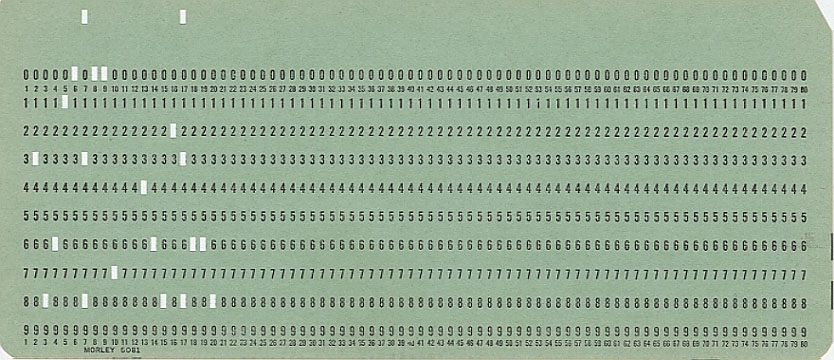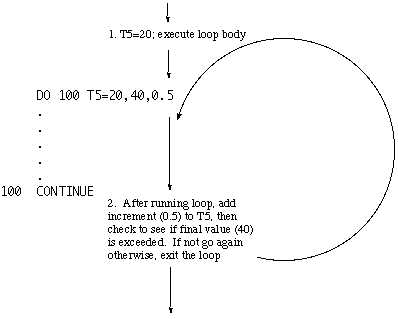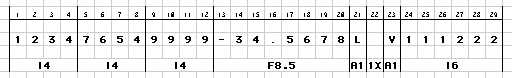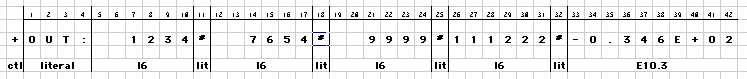FORTRAN IV Reference Page
Programming Languages, Gordon College

```
Program Organization

All FORTRAN programs have the following format:

PROGRAM statement
variable declarations (optional)
executable statements
END statement
SUBROUTINE or FUNCTION modules (optional)

The original FORTRAN programs were prepared on a keypunch machine which punched holes into paper
cards which had 80 characters maximum.  For this reason, lines in a FORTRAN program are often
referred to as "cards."  Each card is either a "data" card, a "comment" card or a "statement" card.

``````
Data Cards
All 80 characters of the line are used for program data as defined below.

Comment Cards
The first character on the card much be C; all other characters are ignored in subsequent
processing.

Statement Cards
Statement cards are subdivided into four sections as follows:

1         2         3         4         5         6         7            8
12345 | 6 | 789012345678901234567890123456789012345678901234567890123456789012 | 34567890

The first five characters are used for unique statement numbers.  Numbers do not need
to appear in sequence.  Any statement (except the END statement) may have a statement
number.
The sixth character is called the "continuation" character.  If more space is required
from the previous card, include any character (except 0) in the 6th position of the next card.
Positions 7-72 are used for the actual program code.  Often programmers use a TAB (8 spaces)
rather than type 7 spaces.
Positions 73-80 are infrequently used, but when they are they are used for identification
codes which are only of interest to the programmer, they are not computed.

Character set
(ASA Standard FORTRAN IV)

A .. Z
0 .. 9
=
+
-
*
/
(
)
,
.
\$

Note: All modern versions of FORTRAN support more characters than these.

Identifiers
While FORTRAN is very picky about the placement of certain program elements on a card, it is
supremely disinterested otherwise.  For example, the keyword PROGRAM, which begins a FORTRAN
program, may be typed,

PROGRAM
PRO GRAM
PR OG RAM

or even,

P R  O    G     R      A       M

This is partly due to the difficulty in repairing a typographical error when using a keypunch
machine.  Likewise, whitespace is not required between syntactic objects in a program.  In other
words the FORTRAN executable statement,

DO 30 I = 10, 100

can also be written,

DO30I=10,100

Such forms can lead to serious errors.  Imagine, for example, what would have occurred if the
programmer accidentally punched a decimal point instead of a comma in the compressed example
above.

Reserved words
FORTRAN has no reserved words.  All words have meaning based on context.  For example, the
following is legal, though unwise, in FORTRAN

IF (IF.EQ.THEN) IF=IF*THEN

where the first use of the word IF refers to the keyword for a selection control structure,
while the other uses refer to a variable named IF; likewise, THEN is used in the context
of a variable in this statement.

Data types and Variables

Variable declaration

Variables are named through user-defined identifiers; however, they do not need to be
explicitly declared in a FORTRAN program.  Variables are declared either implicitly, by use
of the variable's name or explicitly through a "type" statements, either DATA or DIMENSION.

Any variable beginning with the letters I .. N is implicitly declared to
be an INTEGER data type (see below) unless overridden by an explicit
declaration.  All other variables are implicitly declared to be REAL data
type (see below) unless overridden.

Declaration statements require type name, followed by list of identifiers, for example:

INTEGER DAY,WEEK,MONTH
REAL IM67,QU45
LOGICAL DONE

Data types

Primitive

Numeric types

INTEGER      literal examples:     0
42
-12
+2345
0034

REAL                 literals:     0.
1.1
-.234
+12.34
1.234E-12

DOUBLE PRECISION     literals:     13.D+1
13D1

COMPLEX              literals:     CMPLX(1,2)              means    1 + 2i
CMPLX(+12739E3,0.)
CMPLX(44.36,-12.2E16)

Boolean type

LOGICAL              literals:     .TRUE.
.FALSE.

String type

CHARACTER            literals:     4HJOHN
6HR CASH
'JOHNNY CASH'   -- note, not ASA standard

User-defined

FORTRAN IV does not support the creation of a user-defined data type

Structured Data

Arrays

Arrays are created with DIMENSION statements and may have 1 to 7 subscripts
(depending upon implementation.

DIMENSION W(1000)
DIMENSION X(10,10)
DIMENSION Z(4,6,8,10)

or

DIMENSION W(1000),X(10,10),Z(4,6,8,10)

Note that the variable arrays above will contain REAL data because no explicit
data type was declared.  To explicitly declare the type of value in the array use
the form, for example,

DOUBLE PRECISION A,K,M(5,5,10),X

which will create three unstructured double precision variables (A, K and X) as
well as an array of 250 double precision values (M).

FORTRAN stores arrays in column-major order meaning that the earlier subscripts
vary most rapidly in the actual stored data.  For example, X(1,2) is followed in
memory  by X(2,2) not X(1,3).  Alternately, values can be referenced by variable
subscripts such as X(K) or X(K+1); note the use of an integer variable subscript.

Records

FORTRAN IV does not support record structures.

Variable initialization

DATA statements are used to initialize variables.  Data statements begin with the
keyword DATA and are followed by identifiers and values, delimited by slashes.
For example, either

DATA I,J,K/0,0,0/

or

DATA I,J,K/3*0/

results in the following:

I0

J0

K0

while

DIMENSION L(2,3)
DATA L/1,2,3,4,5,6/

results in

L(1,1)L(1,2)L(1,3)
135
L(2,1)L(2,2)L(2,3)
246

Note that L is implicitly an array of integers.  Also, notice the
effect of column-major order.

Assignment Statements

Assignments are made as follows,

variable = expression

where the variable is either implicitly or explicitly declared and the expression is either a
literal value (e.g. T=42.) or a computed value (e.g. I=K+L), as described below.

Expressions

Numeric

OPERATION        OPERATOR     ORDER OF PRIORITY

group              (  )              1
exponentiate        **               2
multiply            *                3
divide              /                3 (see note)
subtract            -                4

Note:  type of operands affects result.  For example,

10/4  evaluates to 2
10./4 evaluates to 2.5

Type conversion and coercions

In general, mixed mode math is discouraged in FORTRAN.  When using operands with
different types, automatic conversion occur as follows:

if any operand is COMPLEX, result is COMPLEX
else
if any operand is DOUBLE PRECISION, result is DOUBLE PRECISION
else
if any operand is REAL, result is REAL
else
result is INTEGER

To convert from one data type to another explicitly, many built-in functions are
provided.  For example,

Conversion          ------------------ Function Result Type ---------------------
Functions

From:          To:     INTEGER          REAL         DOUBLE PRECISION     COMPLEX
--------------   ------------    ----------------     -------

REAL                   INT()           AINT()1             DBLE()         CMPLX(A,B)2
IFIX()          ANINT()
NINT()3

INTEGER                                FLOAT()

DOUBLE PRECISION       IDINT()1         SNGL()
IDNINT()1

COMPLEX                                REAL()
AIMAG()

1 Truncates the real number.  Same as: FLOAT(INT())
2 Creates complex number A+Bi
3 "NINT" functions round to the nearest integer

Logical

OPERATION                      OPERATOR     ORDER OF PRIORITY

comparison                                   (after numeric)

less than                    .LT.              5
less than or equal to        .LE.              5
equal to                     .EQ.              5
not equal to                 .NE.              5
greater than or equal to     .GE.              5
greater than                 .GT.              5

Boolean

not                          .NOT.             6
and                          .AND.             7
or                           .OR.              8

Character

No character manipulations were possible in FORTRAN IV; however, FORTRAN 77 included
many of these useful features.

Function call

FORTRAN's many built-in functions evaluate as expressions.  User-defined functions (see
below) likewise are called as expressions.  Below are some of the more commonly used
functions of FORTRAN IV.

Function            ---------- Function Result Type / Parameter Type-------------

INTEGER          REAL           DOUBLE PRECISION     COMPLEX
--------------   ------------    ----------------     -------
absolute value        IABS()          ABS()              DABS()
square root                           SQRT()             DSQRT()          CSQRT()
remainder of A/B      MOD(A,B)        AMOD(A,B)          DMOD(A,B)
e**x                                  EXP()              DEXP()           CEXP()
natural log                           ALOG()             DLOG()           CLOG()
base 10 log                           ALOG10()           DLOG10()
sine                                  SIN()              DSIN()           CSIN()
cosine                                COS()              DCOS()           CCOS()
arctangent                            ATAN()             DATAN()
maximum of list       MAX0(A,B,...)   AMAX1(A,B,...)     DMAX1(A,B,...)
minimum of list       MIN0(A,B,...)   AMIN1(A,B,...)     DMIN1(A,B,...)
positive difference
= A-MIN(A,B)        IDIM(A,B)       DIM(A,B)
sign transfer
= ABS(A)*sign of B  ISIGN(A,B)      SIGN(A,B)          DSIGN(A,B)

Note: In this table, unless more than one parameter is explicitly demonstrated
above, all functions require one parameter which is an expression
of the indicated type.

When 2 or more parameters are required, they are separated by commas.

Some functions have results whose type differ from the parameters.  The conversion
functions above are the prime examples.  In addition, FORTRAN IV has the following
functions:

INTEGER maximum from list of REAL values        MAX1(A,B,...)
INTEGER minimum from list of REAL values        MIN1(A,B,...)
REAL maximum from list of INTEGER values        AMAX0(A,B,...)
REAL minimum from list of INTEGER values        AMIN0(A,B,...)

Control Statements

Branch

GO TO 1005                  ;   unconditional branch
go directly to statement number 1005

GO TO K                     ;   assigned branch
go to statement number whose value is stored in K

GO TO (10,20,1000,20),K     ;   computed branch
K=1, 2, 3 or 4 branches to statement number 10, 20, 1000 or 20

Selection

Logical IF

IF (logical-expression) any valid statement except DO or IF

Evaluate the expression, then execute the statement only if the logical-expression
was .TRUE.  For example,

IF (X.GT.T*Z) Y=X/FLOAT(K)

Arithmetic IF

IF (numeric-expression) statement1,statement2,statement3

Evaluate the expression, then transfer to statement1 if the result is negative, to
statement2 if zero, to statement3 if positive.  For example,

IF (X/Y*Z) 100,300,50

If the result of the computation is negative, transfer to statement number 100, if zero
transfer to statement number 300, if positive to statement number 50.  Note that easrly
FORTRAN had no block structure like the familiar { ... } or BEGIN ... END found
in many other languages.  Block structure can be simulated as follows, using a
Java-like snippet for comparison.

Block-structure        Unstructured FORTRAN workaround
---------------        -------------------------------
if (i == 42)                   IF (I.NE.42) GOTO ###
{                              STMT-1
stmt-1;                    STMT-2
stmt-2;                    STMT-3
stmt-3              ###    ≤ next statement goes here >
}

Repetition

Regular DO

DO statement#  variable = initialValue, finalValue, optionalIncrement

Do this statement and all other statements upto the card labelled with statement#, beginning
by setting the variable to the initialValue, then incrementing by adding 1 (or the
non-negative optional increment value) with each successive iteration until
variable > finalValue.  Once the loop is finished, proceed to the next card
beyond the labelled statement#.

Note:  Branching out of a DO loop body is allowed, but branching in is not.
Post-test loop; always executed at least once.
``````
Nested DO

Unlike the IF statement, FORTRAN allows nesting of any number of DO loops within one
another.  The general form of a nested loop is,

DO stmt#1 variable1 = start, finish, step
.
.
.
DO stmt#2 variable2 = start, finish, step
.
.
.
stmt#2     CONTINUE
.
.
stmt#1 CONTINUE

Modularity

Functions

Functions may be used anywhere that an expression is expected in a FORTRAN program.
As described above, a large number of functions are provided in the standard FORTRAN
library.  These built-in functions are referred to as intrinsic functions.

User-defined

Modern versions of FORTRAN have a much broader collection.  Many books have
been published with useful FORTRAN functions which are not available in the standard
library. The programmer implements functions using the syntax,

optResultType FUNCTION name(formalParamList)
optFormalParameterDeclaration
optLocalVarDeclaration
executableStatements
name = value
RETURN
END

for example,

PROGRAM DEMO
.
.
.
IF (CMPVAL(M1,M2,M3)) GOTO 500
.
.
.
END
C     -------- END OF PROGRAM -------
LOGICAL FUNCTION CMPVAL(I,J,K)
INTEGER SUM, HLFSUM
LOGICAL RESULT
SUM = I+J+K
HLFSUM = SUM/2
RESULT=(I.GT.HLFSUM).OR.(J.GT.HLFSUM).OR.(K.GT.HLFSUM)
CMPVAL=RESULT
RETURN
END
C     -------- END OF FUNCTION -------

Subroutines

When a programmer needs to build a module which returns either 0 or more than 1 result,
a subroutine is used, rather than a function.  Form of the subroutine is similar to the
function,

SUBROUTINE name(formalParamList)
optFormalParameterDeclaration
optLocalVarDeclaration
executableStatements
RETURN
END

A subroutine receives values from the caller through the parameter list; likewise, the
subroutine returns values by modifying the variables in the parameter list.  Subroutines
are invoked in a FORTRAN program as a statement, not an expression.  The syntax used is,

CALL name(actualParamList)

General Limitations

0. Early versions of FORTRAN did not allow recursive function calls.

1. The names chosen for the formal parameters must agree in type with the actual
parameters which will be passed or the data will be coerced to the new type.

2. Use of global variables is not allowed without use of a COMMON statement (discussed
in references).

3.  Subroutines and functions can be thought of as totally separate program units.  For
example, statement numbers which were used in the main program can be reused in the
subprograms.

Input/Output Statements

Data Formats

Due to the nature of the original keypunched FORTRAN, data is highly formatted in a
FORTRAN program.  Consider, for example, a program that needs to process 4 three-digit
integers, a real number, 2 characters, separated by a space and a six-digit integer.

For example,
``````

Input of formatted data

FORTRAN has several codes used to describe different types of data.  For example,

I/O code    Data type for I/O
Iw          INTEGER
Fw.d        REAL
Ew.d        REAL data in exponential form
Dw.d        DOUBLE PRECISION
Aw          ALPHAMERIC (character)
wX          space

where w refers to the total width of the field of data in characters and d refers
to the number of digits after the decimal point.  Input is handled through the use of
numbered FORMAT statements.  For example, to read the data above, one might use,

333    FORMAT(I4,I4,I4,F8.5,A1,1X,A1,I6)

or, alternately,

333    FORMAT(3I4,F8.5,A1,1X,A1,I6)

along with a READ statement,

where unit is the integer associated with the input device.  Standard input device units
are machine specific and might be, for example,

2    magnetic tape
5    keyboard
6    console display
8    card punch

All version of FORTRAN in use today use unit 5 for keyboard input, and unit 6 for output
to the terminal.  Thus, we can read the variables able using,

333    FORMAT(3I4,F8.5,A1,1X,A1,I6)

Output of formatted data

Output is handled much the same as input, with two significant difference: the first
output character is not printed but is used as a control character as specified below.

First character
in Output code       Meaning
Tn                Tab to location n on line
/                 insert blank line
blank             single space
+                 no carriage control
0                 double space line
1                 begin line on new page

Secondly, the format list may include literal strings to be output as well as the variable
values.  For example, we could output the values above using,

334    FORMAT('+OUT:',I6,'#',I6,'#',I6'#',I6,'#',E10.3)
WRITE(6,334) INT1,INT2,INT3,INT4,REAL1

which displays, on the same line as the last output character,

``````

Note that numeric data is right-justified in fields when more space is available.
Character data is left-justified.  Note also that width of the decimal field may
result in a rounded displayed value.

List-directed I/O

Modern version of FORTRAN also allow input and output to be entered via delimited entry.
For example, the same result as above could have been obtained from

where all numeric values will be separated by whitespace in the input stream. Likewise,
an output could be handled as,

WRITE(6,*) ' The output values:',INT1,',',INT2,',',INT3,',',INT4

```

References

• Compaq FORTRAN Language Reference Manual.
• FORTRAN IV POCKET HANDBOOK, Daniel Alexander and Andrew Messer, McGraw-Hill, 1972.
• Programming Languages, Design and Implementation, Terrence Pratt and Marvin Zelkowitz, Prentice Hall, 2001.
• Programming Languages, 2nd ed., Allen B. Tucker, McGraw-Hill, 1986.
• Sample programs:
Sample #1
Sample #2
Sample #3